Sale ends in
Teacher: Kimberly
Customers Who Have Viewed This Course: 2449
\$249.00
\$119.00

#### 0 3rd Grade Curriculum Introduction 01:09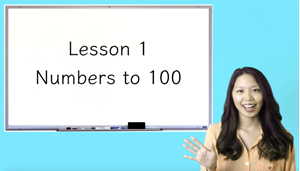This video is an introduction to the 3rd grade curriculum.

#### 1 Lesson 1 Numbers to 100 10:19In this lesson, students will skip count by 1's, 2's, 5's, and 10's, count base 10 blocks, sort 2-digit numbers from low to high and high to lower, and sort even and odd numbers.

#### 2 Lesson 1 Worksheet Answer Key 06:19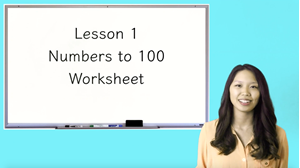This video contains the explanations to the Lesson 1 Numbers to 100 Worksheet.

#### 3 Lesson 2 Addition and Subtraction of 2-Digit Numbers 10:10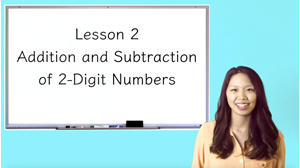In this video, students will add and subtract 2-digit numbers with and without regrouping.

#### 4 Lesson 2 Worksheet Answer Key 05:50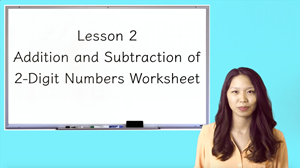This video contains the explanations to the Lesson 2 Addition and Subtraction of 2-Digit Numbers Worksheet.

#### 5 Lesson 3 Numbers to 1000 12:42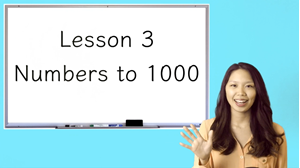In this lesson, students will skip count to 1000, arrange digits to make the smallest or largest possible number, sort 3-digit numbers from low to high and from high to low, and identify the even or odd number that comes before or after.

#### 6 Lesson 3 Worksheet Answer Key 06:22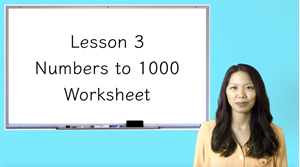This video contains the explanations to the Lesson 3 Numbers to 1000 Worksheet.

#### 7 Lesson 4 Addition and Subtraction of 3-Digit Numbers (Part 1) 10:32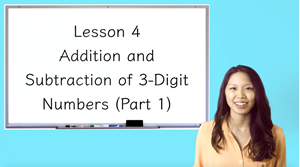In this lesson, students will add and subtract 3-digit numbers with regrouping twice.

#### 8 Lesson 4 Worksheet Answer Key 07:54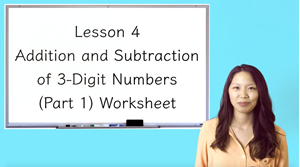This video contains the explanations to the Lesson 4 Addition and Subtraction of 3-Digit Numbers (Part 1) Worksheet.

#### 9 Lesson 5 Addition and Subtraction of 3-Digit Numbers (Part 2) 12:24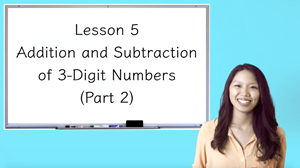In this lesson, students will solve word problems using 3-digit addition and subtraction.

#### 10 Lesson 5 Worksheet Answer Key 10:23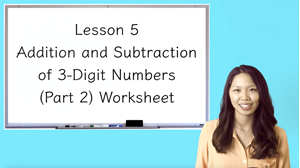This video contains the explanations to the Lesson 5 Addition and Subtraction of 3-Digit Numbers (Part 2) Worksheet.

#### 11 Lesson 6 Length and Distance (Part 1) 15:51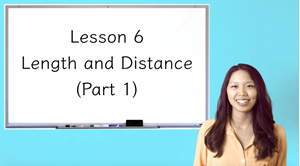In this video, students will use a ruler to measure lengths in whole, half, quarter, eighth and sixteenth inches and convert between inch, feet, and yards.

#### 12 Lesson 6 Length and Distance (Part 1) Worksheet 09:04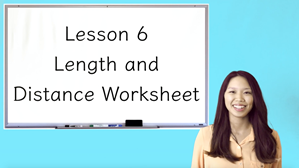This video contains the explanations to the Lesson 6 Length and Distance (Part 1) Worksheet.

#### 13 Lesson 7 Length and Distance (Part 2) 12:13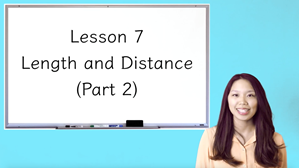In this lesson, students will convert metric to metric lengths and identify the appropriate unit of measurement.

#### 14 Lesson 7 Worksheet Answer Key 06:58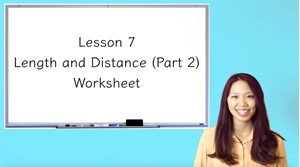This video contains the explanations to the Lesson 7 Length and Distance (Part 2) Worksheet.

#### 15 Lesson 8 Perimeter and Area 14:53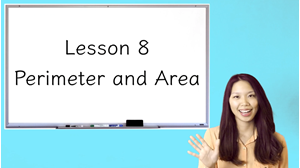In this lesson, students will calculate the perimeter and area of a rectangular figure.

#### 16 Lesson 8 Worksheet Answer Key 09:24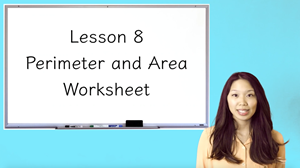This video contains the explanations to the Lesson 8 Perimeter and Area Worksheet.

#### 17 Lesson 9 Time 12:03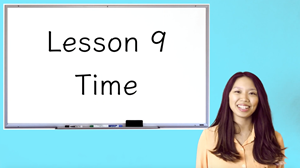In this lesson, students will differentiate between analog and digital clocks, tell time by skip counting and multiplying, and solve word problems to calculate elapsed time.

#### 18 Lesson 9 Worksheet Answer Key 05:49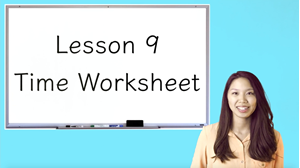This video contains the explanations to the Lesson 9 Time Worksheet.

#### 19 Lesson 10 Money 10:15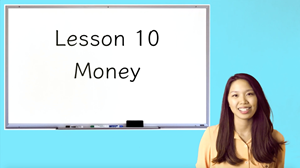In this lesson, students will identify the values of American coins and bills and add American currency.

#### 20 Lesson 10 Worksheet Answer Key 04:18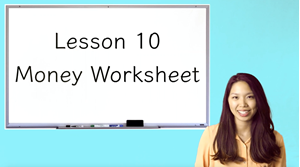This video contains the explanations to the Lesson 10 Money Worksheet.

#### 21 Lesson 11 Addition and Subtraction with Money 11:33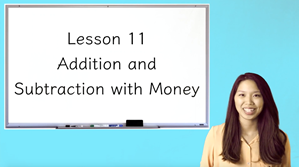In this lesson, students will solve addition and subtraction word problems with money.

#### 22 Lesson 11 Answer Key Worksheet 08:23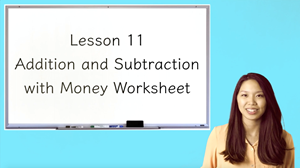This video contains the explanations to the Lesson 11 Addition and Subtraction with Money Worksheet.

#### 23 Lesson 12 Capacity 17:12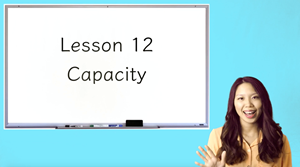In this lesson, students will convert metric to metric liquid volume, convert imperial liquid volume, and identify the appropriate unit of measurement.

#### 24 Lesson 12 Worksheet Answer Key 08:51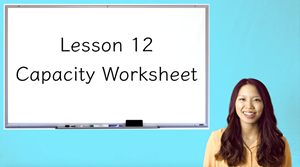This video contains the explanations to the Lesson 12 Capacity Worksheet.

#### 25 Lesson 13 Mass 10:34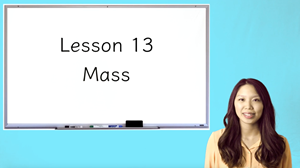In this video, students will convert metric to metric mass and identify the appropriate unit of measurement.

#### 26 Lesson 13 Worksheet Answer Key 07:32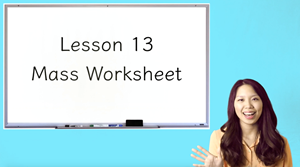This video contains the explanations to the Lesson 13 Mass Worksheet.

#### 27 Lesson 14 Multiplication (Part 1) 10:56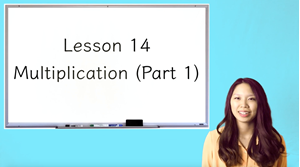In this lesson, students will write multiplication number sentences and apply the Commutative Property, Associative Property and Distributive Property of Multiplication.

#### 28 Lesson 14 Worksheet Answer Key 07:15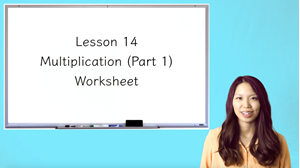This video contains the explanations to the Lesson 14 Multiplication (Part 1) Worksheet.

#### 29 Lesson 15 Multiplication (Part 2) 10:19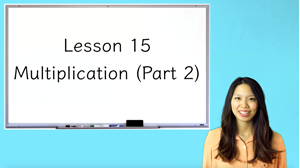In this lesson, students will multiply a 2 digit number and a 1 digit number, as well as, multiply a 3 digit number and a 1 digit number.

#### 30 Lesson 15 Worksheet Answer Key 06:44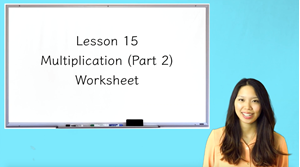This video contains the explanations to the Lesson 15 Multiplication (Part 2) Worksheet.

#### 31 Midpoint Assessment 00:00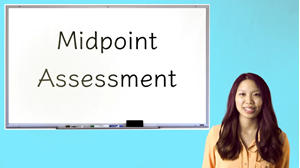Dear Parents,

Please use the attached PDF for your exam, as there are pictures associated with some questions. The answer key can be found in your Parent Resources. The video explanations are in a separate video.

Sincerely,

Teacher Kimberly

#### 32 Midpoint Assessment Answer Key 15:07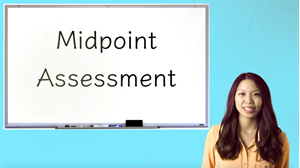This video contains the explanations to the Midpoint Assessment.

#### 33 Lesson 16 Division (Part 1) 13:03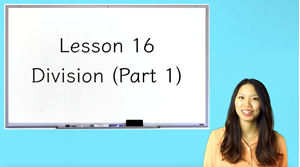In this lesson, students will write division number sentences and write the related multiplication number sentence.

#### 34 Lesson 16 Worksheet Answer Key 09:38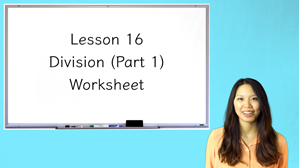This video contains the explanations to the Lesson 16 Division (Part 1) Worksheet.

#### 35 Lesson 17 Division (Part 2) 11:08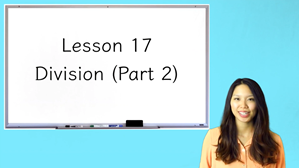In this lesson, students will solve division problems with remainders and solve long division problems using a mnemonic.

#### 36 Lesson 17 Worksheet 09:34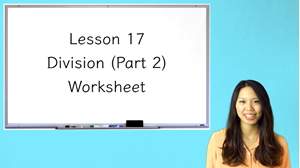This video contains the explanations to the Lesson 17 Division (Part 2) Worksheet.

#### 37 Lesson 18 Multiplication and Division 13:12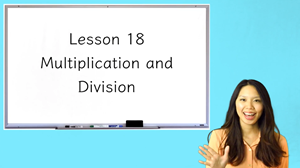In this lesson, students will solve multiplication and division word problems.

#### 38 Lesson 18 Worksheet Answer Key 07:25

This video contains the explanations to the Lesson 18 Multiplication and Division Worksheet.

#### 39 Lesson 19 Fractions (Part 1) 10:26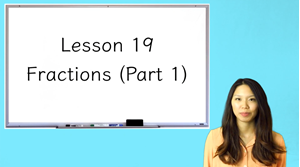In this lesson, students will divide a whole into unit fractions, compare fractions and identify the bigger fraction, and write whole fractions and improper fractions.

#### 40 Lesson 19 Worksheet Answer Key 04:30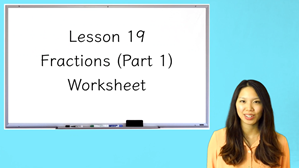This video contains the explanations to the Lesson 19 Fractions (Part 1) Worksheet.

#### 41 Lesson 20 Fractions (Part 2) 17:53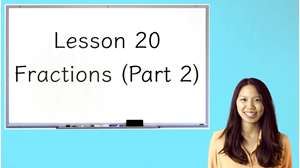In this lesson, students will identify the numerator and denominator of a fraction, label fractions on a number line, find equivalent fractions, compare fractions using the >, < or = symbol, and add similar fractions and mixed numbers.

#### 42 Lesson 20 Worksheet Answer Key 13:59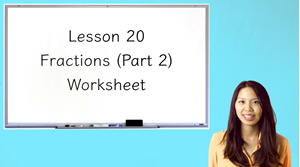This video contains the explanations to the Lesson 20 Fractions (Part 2) Worksheet.

#### 43 Lesson 21 2-D Shapes (Part 1) 13:02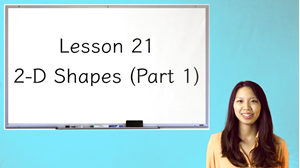Note to parents: I the U.S., that the term “trapezoid” may have two different meanings. In their study, The Classification of Quadrilaterals (Information Age Publishing, 2008), Usiskin et al. call these the exclusive and inclusive definitions:
T(E): a trapezoid is a quadrilateral with exactly one pair of parallel sides
T(I): a trapezoid is a quadrilateral with at least one pair of parallel sides.
These different meanings result in different classifications at the analytic level. According to T(E), a parallelogram is not a trapezoid; according to T(I), a parallelogram is a trapezoid.
Both definitions are legitimate. However, Usiskin et al. conclude, “The preponderance of advantages to the inclusive definition of trapezoid has caused all the articles we could find on the subject, and most college-bound geometry books, to favor the inclusive definition.”

The K-6 Geometry Progression can be found here:
http://commoncoretools.files.wordpress.com/2012/06/ccss_progression_g_k6_2012_06_27.pdf

#### 44 Lesson 21 Worksheet Answer Key 04:24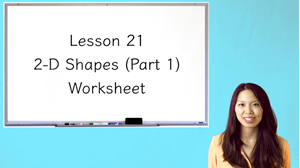This video contains the explanations to the Lesson 21 2-D Shapes (Part 1) Worksheet.

#### 45 Lesson 22 2-D Shapes (Part 2) 11:09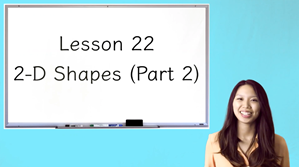In this video, students will find the perimeter of polygons and solve for the length of the missing side(s).

#### 46 Lesson 22 Worksheet Answer Key 07:36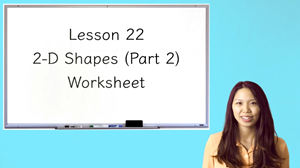This video contains the explanations to the Lesson 22 2-D Shapes (Part 2) Worksheet.

#### 47 Lesson 23 3-D Shapes (Part 1) 09:45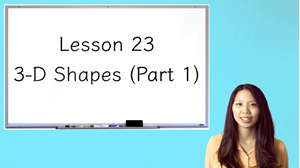In this lesson, students will identify different 3-dimensional shapes, match the 2-D shape with the corresponding 3-D shape and find 3-D shapes in the real world.

#### 48 Lesson 23 Worksheet Answer Key 03:25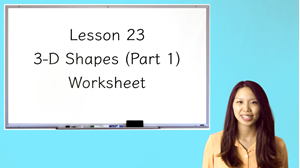This video contains the explanations to the Lesson 23 3-D Shapes (Part 1) Worksheet.

#### 49 Lesson 24 3-D Shapes (Part 2) 12:47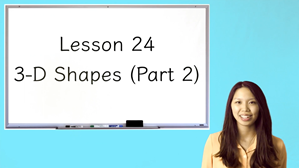In this lesson, students will identify the faces of a 3-D shape, identify the edges on a 3-D shape, and identify the vertices on a 3-D shape.

#### 50 Lesson 24 Worksheet Answer Key 05:16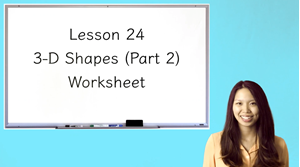This video contains the explanations to the Lesson 24 3-D Shapes (Part 2) Worksheet.

#### 51 Lesson 25 Transformations 12:49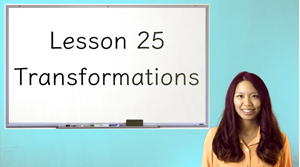In this lesson, students will define the 3 transformations and translate, rotate, and reflect an image.

#### 52 Lesson 25 Worksheet Answer Key 03:14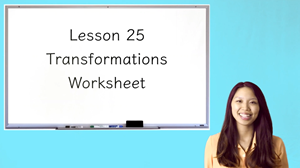This video contains the explanations to the Lesson 25 Transformations Worksheet.

#### 53 Lesson 26 Patterns 12:46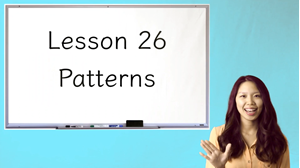In this lesson, students will identify the pattern in a number series and find the missing output in a number pattern.

#### 54 Lesson 26 Worksheet Answer Key 08:51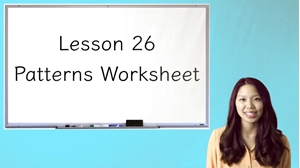This video contains the explanations to the Lesson 26 Patterns Worksheet.

#### 55 Lesson 27 Order of Operations 10:04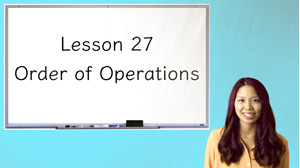In this lesson, students will recite the mnemonic "My Dear Aunt Sally" and solve problems with a combination of operations in the appropriate order.

#### 56 Lesson 27 Worksheet Answer Key 06:56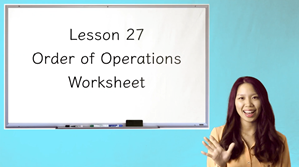This video contains the explanations to the Lesson 27 Order of Operations Worksheet.

#### 57 Lesson 28 Tally Charts and Pictographs 09:50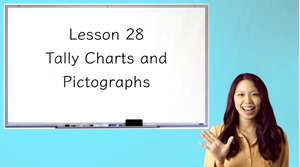In this lesson, students will interpret data in a tally chart and interpret data in a pictograph.

#### 58 Lesson 28 Worksheet Answer Key 10:31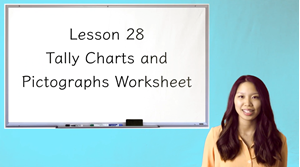This video contains the explanations to the Lesson 28 Tally Charts and Pictographs Worksheet.

#### 59 Lesson 29 Bar Graphs and Line Plots 11:36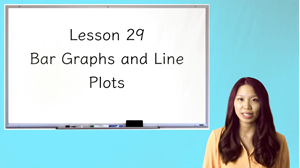In this video, students will interpret data in a bar graph and interpret data in a line plot.

#### 60 Lesson 29 Worksheet Answer Key 08:22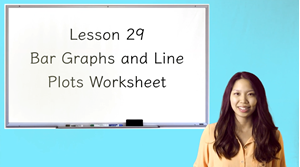This video contains the explanations to the Lesson 29 Bar Graphs and Line Plots Worksheet.

#### 61 Lesson 30 Probability 10:33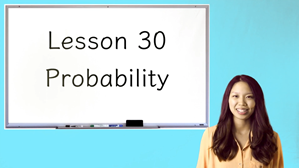In this lesson, students will calculate the probability of an event.

#### 62 Lesson 30 Worksheet Answer Key 03:51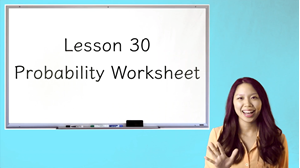This video contains the explanations to the Lesson 1 Numbers to 100 Worksheet.

#### 63 Yearly Assessment 00:00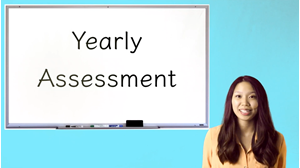Dear Parents,

Please use the attached PDF for your exam, as there are pictures associated with some questions. The answer key can be found in your Parent Resources. The video explanations are in a separate video.

Sincerely,

Teacher Kimberly

#### 64 Yearly Assessment Answer Key 17:57This video contains the explanations to the Yearly Assessment.

Welcome to my 3rd grade classroom!

This engaging math course contains:

• 11 sections
• 30 lessons
• 30 worksheet counterparts
• 30 worksheet review videos (I review each worksheet problem one by one!)
• Midpoint Assessment
• Yearly Assessment
• Parental worksheet and assessment answer keys

This course has been designed to closely mimic the Common Core curriculum, but also covers additional topics that all 3rd graders need to know.

After Lesson 15, there is a Midpoint Assessment that test the skills the students have accumulated thus far. The Midpoint Assessment Explanation Video also contains the corresponding lesson topic for each question. If you need to review a question, the topic will be easy to identify and find.

After Lesson 30, there is a Yearly Assessment that combines all the skills that the students have acquired from the entire year.

Overview:

We begin the course with a basic review to ensure a strong foundation. These skills include counting to 1000, addition and subtraction of 3 digit numbers, as well as reading a ruler. As the course progresses, students begin to work with 2 and 3-dimensional shapes, calculate perimeter and area, fractions, as well as multiplication and long division. By the end of the course, students will have been exposed to numerous word problems that test their command and understanding of arithmetic operations. This course will help prepare you for the 4th grade!

Course Topics:

Unit 1: Basics: Counting, Addition, and Subtraction

• Students will skip count by 1's, 2's, 5's, and 10's, count base 10 blocks, sort 2-digit numbers from low to high and high to lower, and sort even and odd numbers.
• Students will add and subtract 2-digit numbers with and without regrouping.
• Students will skip count to 1000, arrange digits to make the smallest or largest possible number, sort 3-digit numbers from low to high and from high to low, and identify the even or odd number that comes before or after.
• Students will add and subtract 3-digit numbers with regrouping twice.
• Students will solve word problems using 3-digit addition and subtraction.

Unit 2: Measurement

• Students will use a ruler to measure lengths in whole, half, quarter, eighth and sixteenth inches and convert between inch, feet, and yards.
• Students will convert metric to metric lengths and identify the appropriate unit of measurement.
• Students will calculate the perimeter and area of a rectangular figure.
• Students will differentiate between analog and digital clocks, tell time by skip counting and multiplying, and solve word problems to calculate elapsed time.
• Students will identify the values of American coins and bills and add American currency.
• Students will solve addition and subtraction word problems with money.
• Students will convert metric to metric liquid volume, convert imperial liquid volume, and identify the appropriate unit of measurement.
• Students will convert metric to metric mass and identify the appropriate unit of measurement.

Unit 3: Multiplication

• Students will write multiplication number sentences and apply the Commutative Property, Associative Property and Distributive Property of Multiplication.
• Students will multiply a 2 digit number and a 1 digit number, as well as, multiply a 3 digit number and a 1 digit number.

Unit 4: Midpoint Assessment

Unit 5: Division

• Students will write division number sentences and write the related multiplication number sentence.
• Students will solve division problems with remainders and solve long division problems using a mnemonic.
• Students will solve multiplication and division word problem

Unit 6: Fractions

• Students will divide a whole into unit fractions, compare fractions and identify the bigger fraction, and write whole fractions and improper fractions.
• Students will identify the numerator and denominator of a fraction, label fractions on a number line, find equivalent fractions, compare fractions using the >, < or = symbol, and add similar fractions and mixed numbers.

Unit 7: Shapes and Patterns

• Students will name different sided polygons, identify right angles in polygons, and identify parallel sides in polygons.
• Students will find the perimeter of polygons and solve for the length of the missing side(s).
• Students will identify different 3-dimensional shapes, match the 2-D shape with the corresponding 3-D shape and find 3-D shapes in the real world.
• Students will identify the faces of a 3-D shape, identify the edges on a 3-D shape, and identify the vertices on a 3-D shape.
• Students will define the 3 transformations and translate, rotate, and reflect an image.
• Students will identify the pattern in a number series and find the missing output in a number pattern.

Unit 8: Order of Operations

• Students will recite the mnemonic "My Dear Aunt Sally" and solve problems with a combination of operations in the appropriate order.

Unit 9: Graphs

• Students will interpret data in a tally chart and interpret data in a pictograph.
• Students will interpret data in a bar graph and interpret data in a line plot.

Unit 10: Probability

• Students will calculate the probability of an event.

Unit 11: Yearly Assessment

• Teacher: Kimberly
• Areas of expertise: Mathematics
• Education: Bachelor's Degree in Elementary Education and Mathematics from Queens College, CUNY, NYS Certified Elementary Education License, and TESOL Certificate from Arizona State University
• Interests: I am a language enthusiast and love to travel to different countries.
• Skills:
• Associations: Member of the TESOL International Association

I have over 10 years of experience teaching children from Kindergarten through the 10th grade! I have taught in a classroom setting, given one-on-one individual instruction, and currently teach online to an international community.

For Parents

For Parents

For Parents

For Parents

For Parents

For Parents

For Parents

For Parents

For Parents

For Parents

For Parents

For Parents

For Parents

For Parents

#### Lesson 15 Worksheet Answer Key

For Parents

For Parents, After Lesson 15

For Parents

For Parents

For Parents

For Parents

For Parents

For Parents

For Parents

For Parents

For Parents

For Parents

For Parents

For Parents

For Parents

For Parents

#### Lesson 30 Worksheet Answer Key

For Parents

For Parents, After Lesson 30
Customers who bought this item also boughtIn this course you will learn all about how to use proper grammar in writing and also in everyday speaking. This course is geared towards second graders as they will be learning how to formulate sentences using correct grammar. This course would also be great review for third graders as well. +
\$220.00 \$105.00In this 2nd and 3rd grade comprehensive literacy course, students will be systematically guided through learning and applying the 5 important pillars of reading; comprehension, vocabulary, fluency, phonics, and writing about reading. +
\$229.00 \$109.00This is a fun and engaging complete year's worth of 3rd grade science covering a wide arrange of topics including plants, interdependency of life systems, soil, farming, structures, and forces. +
\$149.00 \$71.00